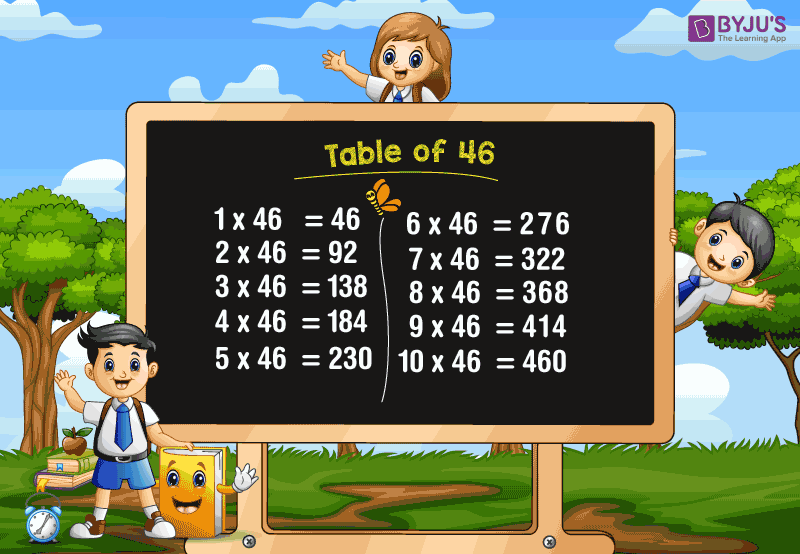# Table of 46

Table of 46 or a multiplication table of forty-six (46) is a bit difficult for the students to memorise quickly. Here, we have given the simple ways of learning 46 times table using chart and table. However, the students who are preparing for competitive exams must learn these Tables from 1 to 100 for quick calculations.

### Download Table of 46 HereWhat is the table of 46?

The table of 46 can be defined using the repeated addition of the number itself, i.e. 46. For example, a class has 3 sections with 46 students each in a school. Here, the total number of students of that class can be calculated using the multiplication table.

Thus, the total number of students = 46 × 3 = 138

This can also be expressed as, 46 + 46 + 46 = 138

Similarly, all the multiples of 46 can be calculated. Also, go through the table of multiplication of forty-six from 1 to 20 given below.

## Multiplication Table of 46

 46 × 1 = 46 46 × 2 = 92 46 × 3 = 138 46 × 4 = 184 46 × 5 = 230 46 × 6 = 276 46 × 7 = 322 46 × 8 = 368 46 × 9 = 414 46 × 10 = 460 46 × 11 = 506 46 × 12 = 552 46 × 13 = 598 46 × 14 = 644 46 × 15 = 690 46 × 16 = 736 46 × 17 = 782 46 × 18 = 828 46 × 19 = 874 46 × 20 = 920

### How to Read the Table of 46

Read the forty-six (46) times table from 1 to 10 as given below.

• One time forty-six is forty six
• Two times forty-six is ninety two
• Three times forty-six is one hundred and thirty eight
• Four times forty-six is one hundred and eighty four
• Five times forty-six is two hundred and thirty
• Six times forty-six is two hundred and seventy six
• Seven times forty-six is three hundred and twenty two
• Eight times forty-six is three hundred and sixty eight
• Nine times forty-six is four hundred and fourteen
• Ten times forty-six is four hundred and sixty

### Get More Multiplication Tables

Get more math tables at www.byjus.com and download BYJU’S – The Learning App to learn maths concepts in an engaging way.

## Frequently Asked Questions on Table of 46

### What is the table of 46?

The table of 46 can be written as:
46 × 1 = 46, 46 × 2 = 92, 46 × 3 = 138, 46 × 4 = 184, 46 × 5 = 230, 46 × 6 = 276, 46 × 7 = 322, 46 × 8 = 368, 46 × 9 = 414, 46 × 10 = 460, etc.,

### Is 46 in the 3 times table?

No, since the numbers that exist in the multiplication table of 3 are 3, 6, 9, 12, 15, 18, 21, 24, 27, 30, 33, 36, 39, 42, 45, 48, 51,…

### What equals 46 in multiplication?

The number 46 can be represented as the multiplication as given below.
46 = 1 × 46
2 × 23 = 46
Or
46 × 1 = 46
23 × 2 = 46

### What is the table of 48?

The table of 48 is given as:
48 × 1 = 48, 48 × 2 = 96, 48 × 3 = 144, 48 × 4 = 192, 48 × 5 = 240, 48 × 6 = 288, 48 × 7 = 336, 48 × 8 = 384, 48 × 9 = 432, 48 × 10 = 480, etc.,

### What is the table of 42?

The table of 42 is given below from 1 to 10 times.
42 × 1 = 42, 42 × 2 = 84, 42 × 3 = 126, 42 × 4 = 168, 42 × 5 = 210, 42 × 6 = 252, 42 × 7 = 294, 42 × 8 = 336, 42 × 9 = 378, 42 × 10 = 420